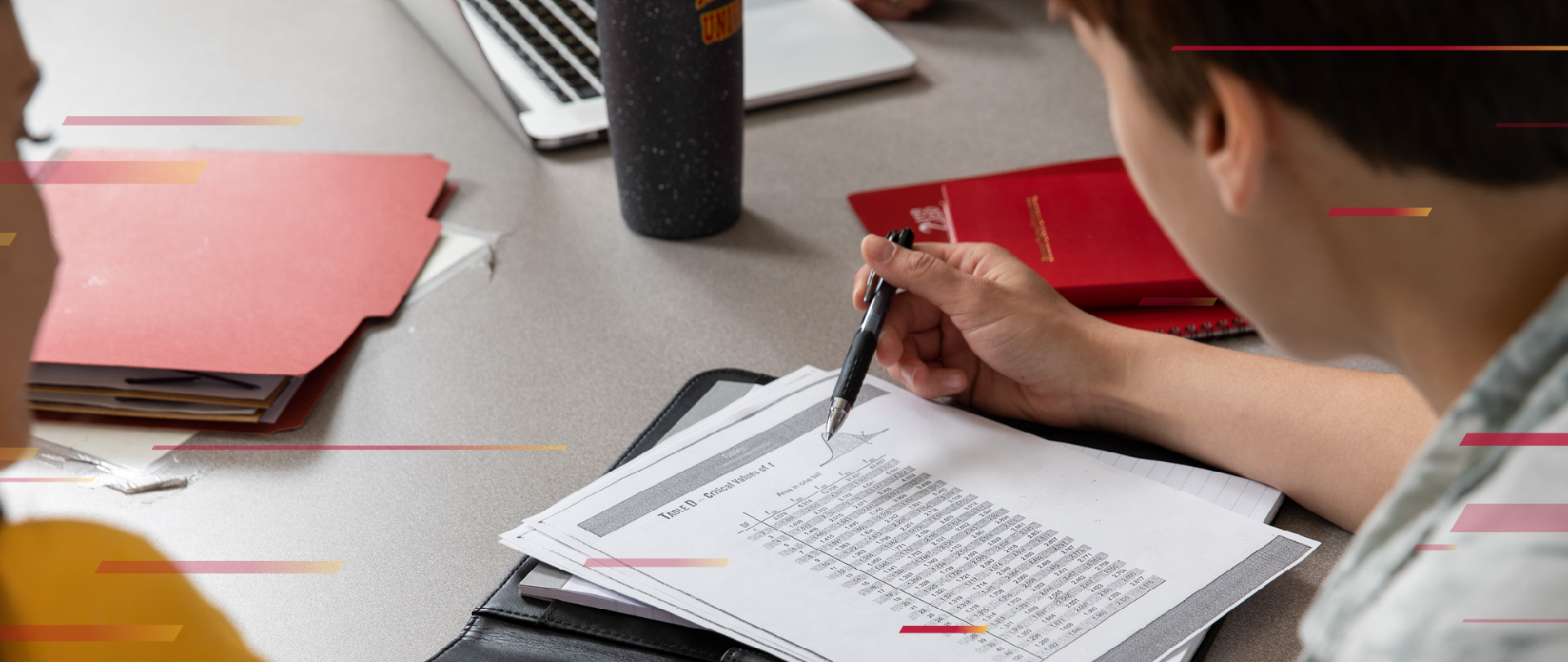# Applied Mathematics

Options: Bachelor's

Locations: Main Campus

## General Information

The Applied Mathematics bachelor's degree is designed for students who want a broad knowledge of mathematics and its many types of applications. Courses in statistics, operations research, and computer science can be combined for a well-rounded applied mathematics foundation, which can lead to careers in business and industry, as well as entrance into graduate programs.

• 2.50 High School GPA (on a 4.00 scale)
• Minimum Composite ACT Score of 17/SAT 900
• High school courses and grade point average, ACT composite score, and ACT Reading and Mathematics subscores will be considered in the admission and placement process.

• 2.00 Overall GPA (on a 4.00 scale)
• At least 12 credits, including an English and Mathematics course.

## Required Courses

CPSC 130 Programming and Problem Solving (4)
CPSC 200 Object Oriented Programming (4)
MATH 220 Calculus 1 (4)
MATH 230 Calculus 2 (4)
MATH 251 Statistics (3)
MATH 320 Calculus 3 (4)
MATH 322 Linear Algebra (3)
MATH 324 Fundamental Concepts in Mathematics (3)
MATH 330 Differential Equations (3)
MATH 340 Numerical Analysis (3)

Electives (select 5 from the following: CPSC 300, CPSC 320, CPSC 330, CPSC 340, MATH 328, MATH 360, MATH 414, MATH 416, MATH 420, MATH 430, MATH 440)

CPSC 130
MATH 220

CPSC 200
MATH 230

MATH 322
MATH 330

MATH 251
MATH 320

CPSC or MATH
MATH 324

CPSC or MATH
MATH 340

CPSC or MATH

CPSC or MATH

CPSC or MATH